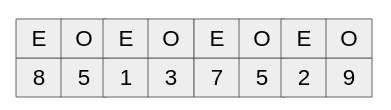## Wednesday, June 17, 2020

### Squarerooting by Aryabhatta

Here is the squareroot method of Aryabhatta.
भागं हरेदवर्गान्नित्यं द्विगुणेन वर्गमूलेन |
वर्गाद्वर्गे शुद्धे लब्धं स्थानान्तरे मूलम् ||
What above rule implies is, (Having subtracted the greatest possible square from the last odd place and then having written down the square root of the number subtracted in the line of square root), always divide the even place (standing on the right) by twice the square root. Then, having subtracted the square (of the quotient) from the odd place (standing on the right), set down the quotient at the next place (i.e., on the right of the number already written in the line of the square root). This is the square root. (Repeat the process if there are still digits on the right).

In many of the Aryabhatta principles we see even and odd digits. These are the digits of the number counted from left to right with left most being 1. Let's take a number like 85137529 and try to apply above method.The last odd place is 5 but since it is not the last digit, we take last two digits to start the computation. The process in simpler English is as follows.
1. Subtract the greatest possible from the last odd place
2. Divide the even place by twice the square root of the preceding old place
3. Subtract  the square of the quotient from the odd place
4. Repeat the process till we have more digits.

Since the left most digit is a even digit, we start with last two digit.
1. The largest square smaller than that is 81, so quotient is 9. Once we subtract 81 from 85, we get 4
2. Now we bring down 1 and divide the number from twice the quotient, dividing 41 from 18 gets us 36 with quotient of 2.
3. On subtracting 36 from 41 we get 5 and bringing down 3 gives us 53.
4. Now we subtract square of last quotient from this which gives us 53-4 = 49.
5. Now we bring down 7 and divide it by twice the quotient till now which is 92*2 = 184. Dividing 497 from 184 gives us 368 with quotient 2.
6. The subtraction gives us 129, we bring down 5 and subtract 4 which is the square of last quotient.
7. We get 1291, bring down 2 and divide it with twice the current quotient which 922 *2 = 1844 giving us a quotient 7.
8. We get 4, we bring down 9. This gives us 49,  Once we divide 49 from square of last quotient, we get zero and we have no more digits left. This (9227) is our square root.